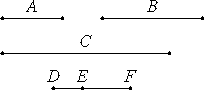# Proposition 15

If three numbers in continued proportion are the least of those which have the same ratio with them, then the sum of any two is relatively prime to the remaining number.

Let A, B, and C, three numbers in continued proportion, be the least of those which have the same ratio with them.

I say that the sum of any two of the numbers A, B, and C is relatively prime to the remaining number, that is, A plus B is relatively prime to C, B plus C is relatively prime to A, and A plus C is relatively prime to B.VIII.2

Take two numbers DE and EF to be the least of those which have the same ratio with A, B, and C.

Cor. to VIII.2

It is then manifest that DE multiplied by itself makes A, and multiplied by EF makes B, and that EF multiplied by itself makes C.

Now, since DE and EF are least, therefore they are relatively prime. But, if two numbers are relatively prime, then their sum is also relatively prime to each, therefore DF is relatively prime to each of the numbers DE and EF.

But, further, DE is also relatively prime to EF, therefore DF and DE are relatively prime to EF. But, if two numbers are relatively prime to any number, then their product is also relatively prime to the other, so that the product of FD and DE is relatively prime to EF, hence the product of FD and DE is also relatively prime to the square on EF.

II.3

But the product of FD and DE is the square on DE together with the product of DE and EF, therefore the sum of the square on DE and the product of DE and EF is relatively prime to the square on EF.

And the square on DE is A, the product of DE and EF is B, and the square on EF is C, therefore the sum of A and B is prime to C.

Similarly we can prove that the sum of B and C is relatively prime to A.

I say next that the sum of A and C is also relatively prime to B.

Since DF is relatively prime to each of the numbers DE and EF, therefore the square on DF is also relatively prime to the product of DE and EF.

II.4

But the sum of the squares on DE and EF together with twice the product of DE and EF equals the square on DF, therefore the sum of the squares on DE and EF together with twice the product of DE and EF is relatively prime to the product of DE and EF.

Taken separately, the sum of the squares on DE and EF together with the product of DE and EF is relatively prime to the product of DE and EF.

Therefore, taken separately again, the sum of the squares on DE and EF is relatively prime to the product of DE and EF.

And the square on DE is A, the product of DE and EF is B, and the square on EF is C.

Therefore the sum of A and C is relatively prime to B.

Therefore, if three numbers in continued proportion are the least of those which have the same ratio with them, then the sum of any two is relatively prime to the remaining number.

Q.E.D.

## Guide

#### Outline of the proof

Let a, b, c be three numbers in continued proportion. Then according to VIII.2, they are of the form

a = d2,     b = de,     c = e2,

where d and e are relatively prime. Then the sum, d + e, is relatively prime to both d and e (VII.28).

Now, since both d and d + e are relatively prime to e, so is their product d2 + de relatively prime to e (VII.24), and therefore to e2 (VII.25). Thus, a + b is relatively prime to c.

Likewise, b + c is relatively prime to a.

Next, since d + e is relatively prime to both d and e, so is its square (d + e)2 relatively prime to the product de (VII.24 and VII.25). That is, d2 + e2 + 2de is relatively prime to de. Subtract 2de to conclude that d2 + e2 is relatively prime to de. Thus, b is relatively prime to a + c. Q.E.D.

This proposition is not used in the rest of the Elements.